# Basic WebGL Meshes

After this session, you will be able to:
• use the mesh primitives of WebGL: points, lines, triangles
• set variables in the shaders from the main program
• apply shader properties uniformly, or locally using data buffers
• use direct or indexed vertex lists

WebGL meshes are drawings based on points in 2d or 3d space, called vertices.
Each vertex is associated with (x,y,z) coordinates, and optionally additional data such as color, surface normal or texture coordinates.
Vertex data is stored in arrays. These arrays are transfered to the graphics hardware and called buffers.

### Points

Example:  Click here to display two points as shown in Figure Two points below.
Question: List the changes to get three points as shown in Figure Three points.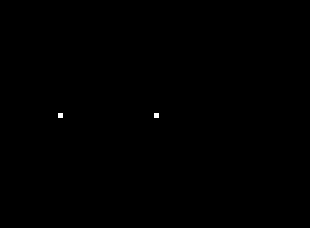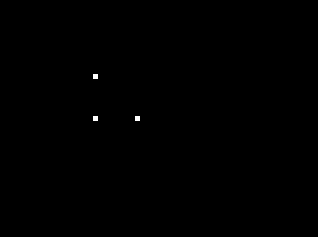Two points Three points Three big points

The vertices are processed using specialized programs written in GLSL language and called vertex shaders, using scripts of type x-shader/x-vertex.
The following shader is used in the previous example. Question: List the changes to draw bigger points as shown in Figure Three big points.
    attribute vec3 aVertexPosition;    uniform mat4 uMVMatrix;    uniform mat4 uPMatrix;    void main(void) {	gl_PointSize = 5.;        gl_Position = uPMatrix * uMVMatrix * vec4(aVertexPosition, 1.0);    }

### Uniform variables

So far, the size of the points is hard coded in the shader. We now want to change it from the main program, based on user input. To do this, we need to:
1. use a variable to represent the point size in the shader
2. get a reference to this variable to access it from the main program
3. use a special method to set the value of this variable
The following page uses a checkbox to switch between point sizes, as illustrated below.
Question: list the program lines used to implement the three steps presented above.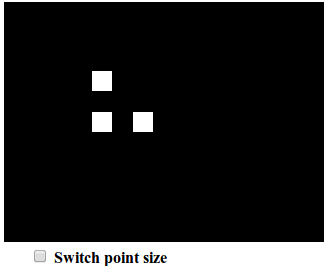Switching between point sizes using a checkbox

Question: replace the checkbox with a slider or a text field to precisely tune the point size.

We now want to use different colors. They are set in the fragment shader script,  with type x-shader/x-fragment, which is used to set the color of each pixel of the final image.
Question: Use the following code line to display the points in yellow:
gl.uniform4fv(shaderProgram.uColor, [1,1,0,1] );

### Lines

This page displays lines using the gl.LINES drawing mode, as illustrated below.
Question: what are the differences with the program which draws three points ?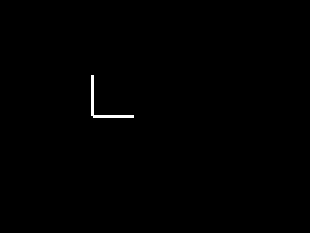Two lines.

There are three line drawing modes, as illustrated below.
Question: how to obtain the two lines shown before, using each of these modes, with the smallest possible number of points ?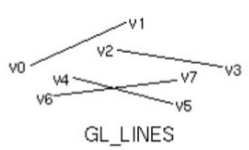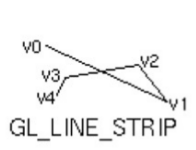### Vertex attributes

We now want a different color for each vertex. To process values on a per-vertex basis, we use an attribute variable instead of a uniform one in the vertex shader. In the following example, a different color was set for each vertex.Points with different colors

Adding color to each vertex requires the following changes:
• In the shader: use an attribute variable per vertex to represent color
• At shader initialization: get a reference to this attribute in the main program, and enable this attribute
• Create a color buffer similar with the position buffer, but with 4 values (for R,G,B,A) per vertex instead of 3
• In the draw function: bind the color buffer to the color attribute
Question: List the program lines used to implement the per-vertex colors.

### Attribute interpolation

In the following example, the color is smoothly interpolated from the different vertices. To allow this, the vertex shader declares and sets a varying variable. This is automatically available to the fragment shader, which uses it to interpolate fragment values between the vertices.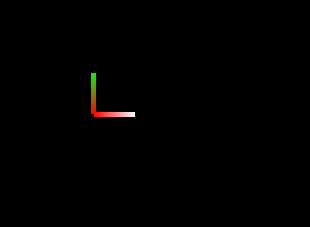gl.LINE_STRIP with color attribute.
Question: List the program lines used to allow color interpolation.

Question: Implement two lines with solid colors, as shown in the following image: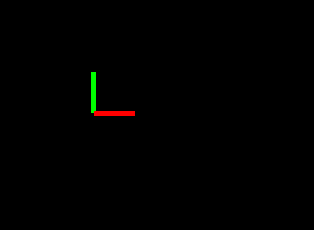Lines with solid color

### Triangles

Triangles can be drawn using one of the three drawing modes illustrated below: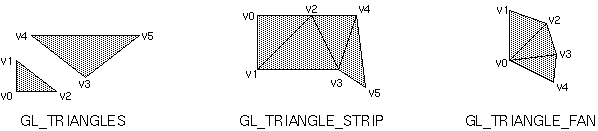Triangle drawing modes

#### Direct vertex lists

This page uses gl.TRIANGLES to draw a cube with one color per face, as illustrated in the following figure. It uses 36 points.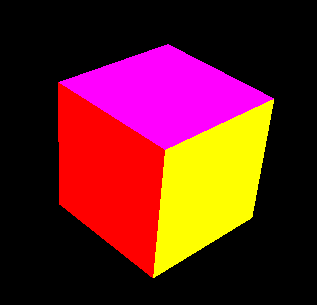Cube with one color per face

#### Indexed vertex lists

This page uses only 8 points, because it uses gl.drawElements instead of gl.drawArrays. Since there is only one color per vertex, the color is interpolated in each face, as illustrated in the following image: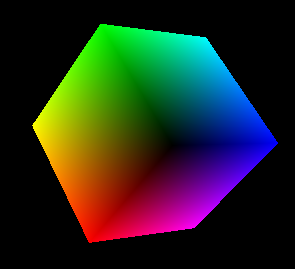Cube with one color per vertex

Question: briefly explain the difference between  gl.drawElements and gl.drawArrays.

Question: modify the previous programs to create a pyramid (5 vertices) with one color per face, and a pyramid with one color per vertex.

### Useful links

Francois Faure, University of Grenoble. Main page